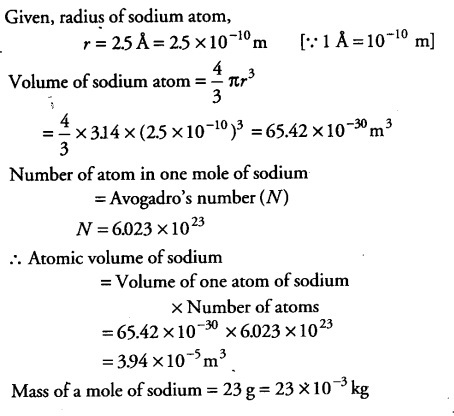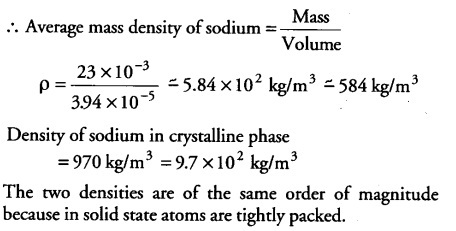# Estimate the average mass density' of sodium atom

Estimate the average mass density’ of sodium atom assuming its size to be about 2.5 A (Use the known values of Avogadro’s number and the atomic mass of sodium). Compare it with the density of sodium in its crystalline phase 970 kg/\${{m}^{3}}\$. Are the two densities of the same order of magnitude? If so, why?